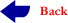MATH 582 -    Nonlinear Programming  (3-0-3)MATH 582 - Course Description

 Assignments number 1 Solution Ass #1 Assignments number 2 Solution Ass#2 Assignments number 3 SolutionAss#3 Assignments number 4 Solution  Ass#4 Solution Q4 Assignments number 5

Syllabus

## Catalog Description

Formulation of linear programs.  Basic properties of linear programs.  The simplex method. Duality. Necessary and sufficient conditions for unconstrained problems.  Minimization of convex functions. A method of solving unconstrained problems.  Equality and inequality constrained optimization.  The Lagrange multipliers theorem.  The Kuhn-Tucker conditions.  A method of solving constrained problems.

Prerequisite:  Math 280; ICS 101, ICS 102, or ICS 103

Objectives

The course deals with the basic ideas of mathematical programming (linear and nonlinear). We shall see how simple mathematics plays a significant role in the development of these ideas. The students will be asked to work out the computational implementation of a numerical algorithm for solving Linear and Nonlinear Program­ming problems and do presentations.

Textbook:  Linear and Nonlinear Programming by E.G. Luenberger, 2nd  edition (1994).

Grading Policy:    First Majaor 15 points, Second Major 15 points, Homework 5 points,  Project 15 points, Final 40 points.

 Week # Sections Topics 1 2.1-2.3 Introduction, Examples of Linear Programming Problems, Basic Solutions 2 2.4-2.5 The Fundamental Theorem of Linear Programming, Relations to Convexity 3 3.1-3.5 Pivots, Adjacent Extreme Points, Determining a Minimum Feasible Solution, Computational Procedure~Simplex Method, Artificial Variables 4 3.7-3.8 Matrix Form of the Simplex Method, The Revised Simplex Method 5 4.1-4.2 Dual Linear Programs, The Duality Theorem 6 4.3-4.5 Relations to the Simplex Procedure, Sensitivity and Complementary Slackness, The Dual Simplex Method 7 5.1-5.4 Transportation Problem 8 6.1-6.4 First Order Necessary Conditions, Examples of Unconstarined Problems, Second-Order Conditions, Convex and Concave Functions, 9 6.5, 7.8 Minimization and Maximization of Convex Functions, Newton’s Method 10 9.1-9.4 Modified Newton’s Method, Construction of the Inverse, Davidon-Fletcher-Powell Method, The Broyden Family 11 10.1-10.3 Constraints, Tangent Plane, First-Order Necessary Conditions 12 13 10.5-10.6, 10.8 Second-Order Conditions, Eigenvalues in Tangent Subspace, Inequality Constraints 14 12.1-12.3 Penalty Methods, Barrier Methods,  Properties of Penalty and Barrier_Functions 15 14.1-14.2,14.4 Quadratic Programming, Direct Methods, Modified Newton’s Methods